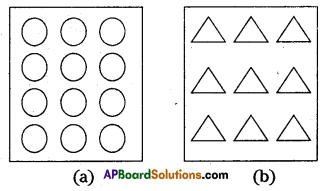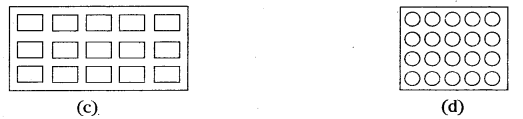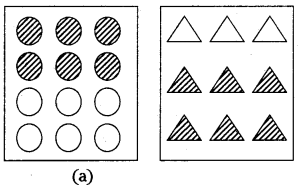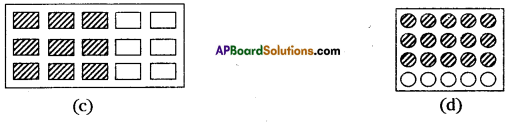# AP Board 7th Class Maths Solutions Chapter 2 Fractions, Decimals and Rational Numbers Ex 2

AP State Syllabus AP Board 7th Class Maths Solutions Chapter 2 Fractions, Decimals and Rational Numbers Ex 2 Textbook Questions and Answers.

## AP State Syllabus 7th Class Maths Solutions 2nd Lesson Fractions, Decimals and Rational Numbers Exercise 2Question 1.
Multiply the following. Write the answer as a mixed fraction.
(i) $$\frac { 3 }{ 6 }$$ x 10
(ii) $$\frac { 1 }{ 3 }$$ x 4
(iii) $$\frac { 6 }{ 7 }$$ x 2
(iv) $$\frac { 2 }{ 9 }$$ x 5
(v) 15 x $$\frac { 2 }{ 5 }$$
Solution:
(i) $$\frac { 3 }{ 6 }$$ x 10 = $$\frac { 30 }{ 6 }$$ = 5 i.e., integer part 5; fraclon part = 0
(ii) $$\frac { 1 }{ 3 }$$ x 4 = $$\frac { 4 }{ 3 }$$ = 1$$\frac { 1 }{ 3 }$$
(iii) $$\frac { 6 }{ 7 }$$ x 2 = $$\frac { 12 }{ 7 }$$ = 1$$\frac {5 }{ 7 }$$
(iv) $$\frac { 2 }{ 9 }$$ x 5 = $$\frac { 10 }{ 9 }$$ = 1$$\frac { 1 }{ 9 }$$
(v) 15 x $$\frac { 2 }{ 5 }$$ = $$\frac { 30 }{ 5 }$$ = 6 i.e., integer part = 6; fraction part = oQuestion 2.
Shade: (i) $$\frac { 1 }{ 2 }$$ of the circles in box (a)
(ii) $$\frac { 2 }{ 3 }$$ of the triangles in box (b)
(iii) $$\frac { 3 }{ 5 }$$of the rectangles in box (c)
(iv) $$\frac { 3 }{ 4 }$$ of the circles in box (d)Solution:Question 3.
Find (i) $$\frac { 1 }{ 2 }$$ of 12 (ii) $$\frac { 2 }{ 5 }$$ of 15
Solution:
(i) $$\frac { 1 }{ 2 }$$ of 12 = $$\frac { 1 }{ 2 }$$ x 12 = $$\frac { 12 }{ 3 }$$ = 4

(ii) $$\frac { 2 }{ 5 }$$ of 15 = $$\frac { 2 }{ 5 }$$ x 15 = $$\frac { 30 }{ 5 }$$ = 6